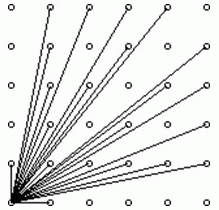Description

作为体育委员，C君负责这次运动会仪仗队的训练。仪仗队是由学生组成的N * N的方阵，为了保证队伍在行进中整齐划一，C君会跟在仪仗队的左后方，根据其视线所及的学生人数来判断队伍是否整齐(如下图)。现在，C君希望你告诉他队伍整齐时能看到的学生人数。共一个数N。

Output

共一个数，即C君应看到的学生人数。

Solutions

其实这题可以转换一下题意。这题等价与用多少条直线可以覆盖所有点。因为所有共线的点中，只有一个能被看到，而且是与原点最近的点

换句话说，对于一条y=kx的直线，只有当$gcd(x,y)=1$时，才能够被看到(对$k$讨论一下就可以得出这样的结论了)。因此，只需要找到所有满足$gcd(x,y)=1$的点对数目即为答案。

用欧拉函数求解即可。$ans=2 \times \sum_{k=1}^{n-1} \phi(k)+ 1$